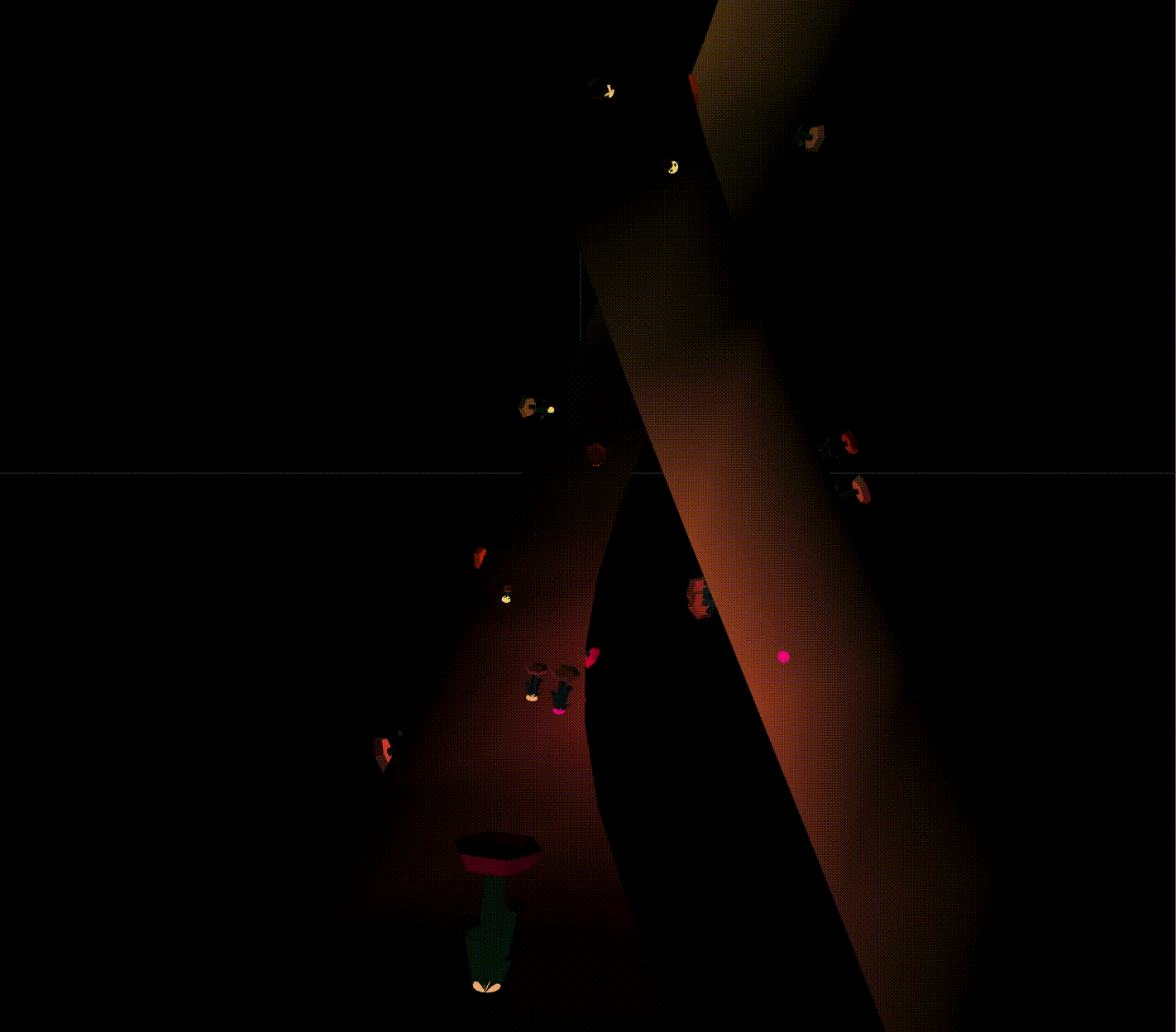## My story

Recently, I have been reading examples in Three.js repository. I dedicate to comprehensive whole the code meanings. After I understand it, I customise the references into my own idea. In this post, I’m going to explain my new visualization that I hit on as a top of the image. The core logic in my code is affected from the previous post that explains an example. What I’m inspired by is the following links.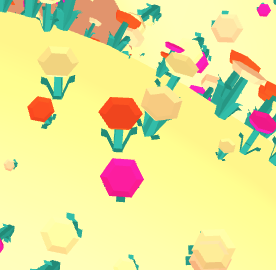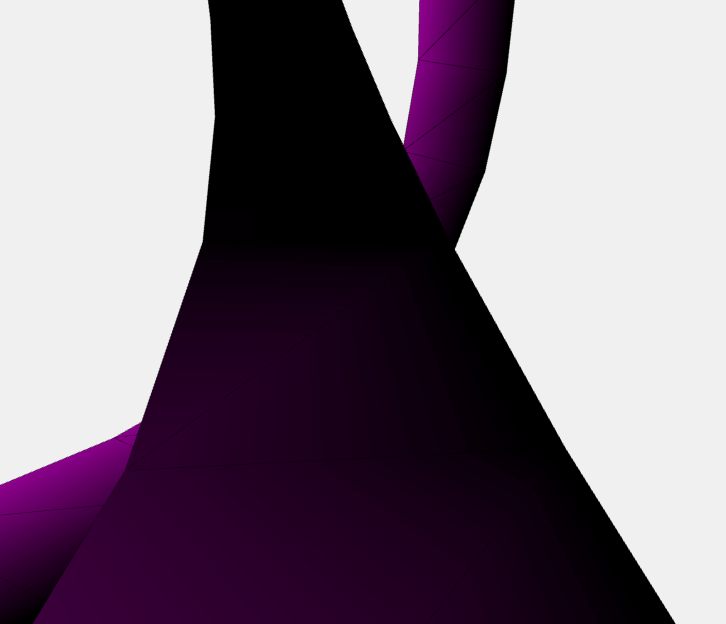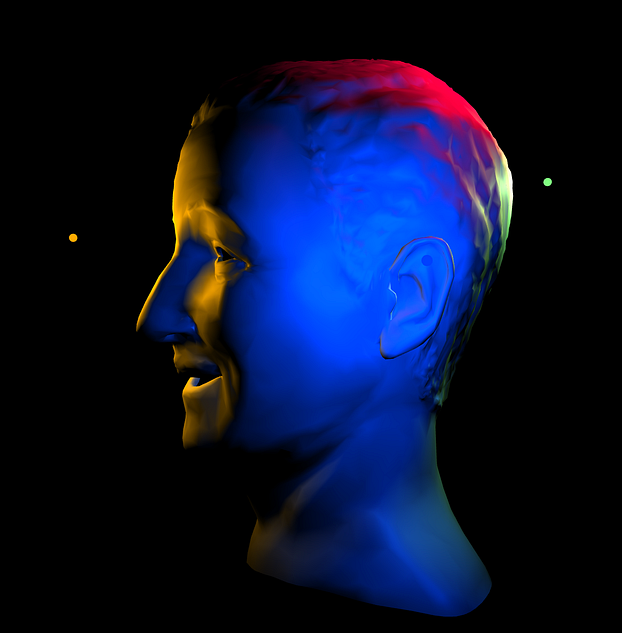Firstly, in accordance with a left example, I chose a couple of code for countless flowers with growing up animation. I have already summarised it in a last-time post. The middle picture demonstrates camera animation along a path. This motion stimulates me. The right side photo above tells how to use a point light in the scene. I came up with eventually going a road alongside flowers.

## Workflow

The mainstream is as follows.

• create a scene, camera, torus geometry
• animate camera along a path on torus geometry
• yield flowers with a point light at the bottom of a stem.

As I explained how to create numerous flowers, I will take the point light and animate camera along the path segmented by geometry.

## Highlight code

#### Point Light

To create a point light is easy. Just call THREE.PointLight with colour. The intensity of the light and how the light spread are optional arguments. As I need the point light at the bottom of every stem of flowers, I create the spotlights as follows.

var blossomPalette = [0xF20587, 0xF2D479, 0xF2C879, 0xF2B077, 0xF24405];
var pointLights;
for (let i = 0; i < count; i++) {
const color = blossomPalette[Math.floor(Math.random() * blossomPalette.length)]
let light = new THREE.PointLight(color, 0.2, 40)
const mesh = new THREE.Mesh(sphere, new THREE.MeshBasicMaterial({ color })
pointLights.push(light)
}

The parent variable above is the container object for a camera as well as geometry and all of the flower objects. After adding the point lights to the scene, set each light on an appropriate flower.

function resampleParticle(i) {
... from previous post
if (pointLights.length > 0) {
const light = pointLights[i]
light.position.x = _position.x * params.scale
light.position.y = _position.y * params.scale
light.position.z = _position.z * params.scale
}
}
• scatter flower is a reference from the previous post, especially in terms of scaling

## Camera Animation

The highlight algorithm for animation according to a path is in getting a current position. We can get the specific position on the path by a method as.getPointAt that is internally Curve object.

var time = Date.now();
var looptime = 20 * 10000;
var t = (time % looptime) / looptime;
surfaceGeometry.parameters.path.getPointAt(t, position);
position.multiplyScalar(params.scale);

We pass tfor a first parameter that requires the range of 0 to 1. Therefore I normalize the value into. The second parameter is indeed optional value but if assigned, returns the copied Vector. See a formal document in detail.

Next to do with is to get a point from tangents. We can get the number of tangents on a geometry by Geometry.tangents, TubuBufferGeometry in this time. As the path point is normalized beforehand, we can specify the segment as follows.

var segments = surfaceGeometry.tangents.length;
var pickt = t * segments;
var pick = Math.floor(pickt);

We gain the current segment on the geometry, we can foreseeable where to move next. At first, calculate the next segment position, then subtract current point from the next point so that camera can translate next position. The image below is the outline of this concept.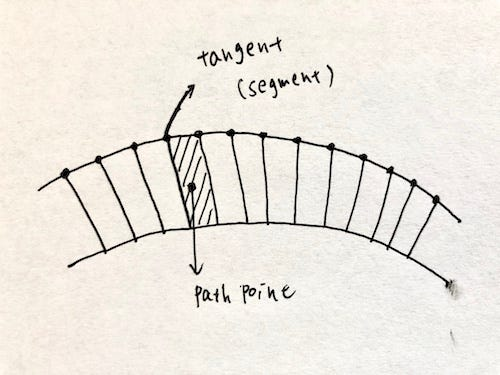The next point where the camera has to slide is the couple of the following code.

var pickNext = (pick + 1) % segments;
binormal.subVectors(surfaceGeometry.binormals[pickNext], surfaceGeometry.binormals[pick]);
binormal.multiplyScalar(pickt - pick).add(surfaceGeometry.binormals[pick]);

We get the next position on the geometry, but the camera direction is not set yet. We can lead the direction by cross product. Calculate it with current tangent and next tangent vector.

surfaceGeometry.parameters.path.getTangentAt(t, direction);
normal.copy(binormal).cross(direction);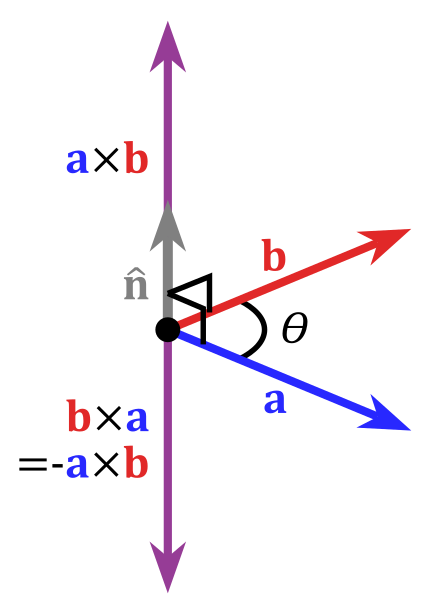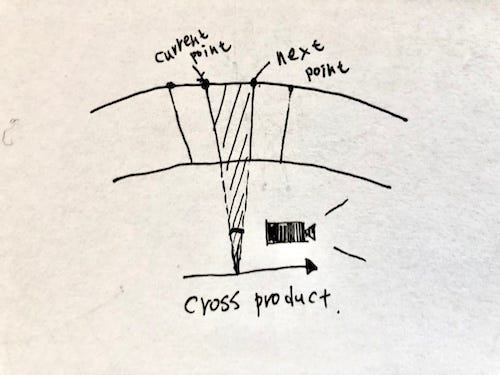Now that we have a proper position, assign it to the camera position and look at the direction.

splineCamera.position.copy(position);
// using arclength for stablization in look ahead
surfaceGeometry.parameters.path.getPointAt((t + 30 / surfaceGeometry.parameters.path.getLength()) % 1, lookAt);
lookAt.multiplyScalar(params.scale);
// camera orientation 2 - up orientation via normal
splineCamera.quaternion.setFromRotationMatrix(splineCamera.matrix);